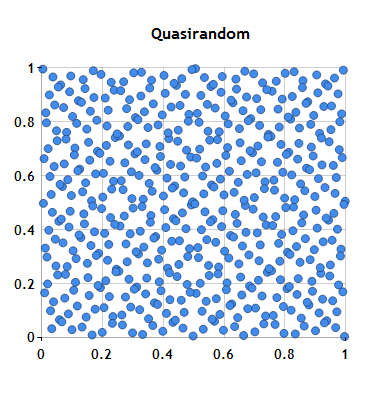# C# Vector Random Numbers

NMath from CenterSpace Software is a .NET class library that provides general vector and matrix classes, complex number classes, numerical integration and differentiation methods, minimization and root finding classes, along with correlation, convolution, and best of class vector random number generators ideal for high performance Monte Carlo simulations.The NMath Core namespace contains a complete framework for generating, streaming, and selecting sets of vector random numbers which supports a large set of distributions.  Additionally, NMath has classes for both Sobel and Niederreiter quasirandom number generators, standard methods for uniformly filling n-space with random numbers. This functionality can be used from any .NET language including VB.NET or F#.

### Random Number Generators Documentation

Complete documentation for all NMath libraries is available online. For general background on the Random Number class library, see the Random Number Generators chapter in the NMath Users's Guide.

• The CenterSpace blog has articles on some aspects of using random number generators with NMath along with code examples.

API documentation for the random number classes is available in the NMath Reference Guide, outlined in the table below. Note that all random number classes are also available in the analogous single precision form.  Compute only with the precision needed as the single precision random number classes can be more that twice as fast as the double precision classes.

Continuous Distributions
Class
Description

Beta distribution.

Cauchy distribution.

Exponential distribution

Gamma distribution.

Gaussian distribution.

Gumbel distribution.

Laplace distribution.

Log-normal distribution.

Rayleigh distribution.

Uniform distribution.

Weibull distribution.
Discrete Distributions

Class
Description
Bernoulli distribution.
Binomial distribution.
Geometric Distribution
Hypergeometric distribution.
Negative Binomial distribution.

Poisson distribution.
Possion distribution with varying mean.
Uniform distribution.
Integer values with uniform bit distribution.

### Random Number Code Examples

All NMath libraries include extensive code examples in both C# and Visual Basic.NET. Studying these examples is one of the best ways to learn how to use NMath libraries. For more information on the NMath vector random number classes, download and try out these examples:

• Example generator vector random numbers [C#]  [VB.NET]
• Example for generating space-filling quasi-random numbers [C#]  [VB.NET]
• Example for generating independent streams of random numbers [C#]  [VB.NET]

### Try a Free EvaluationIf you are interested in evaluating the FFT classes in NMath, we offer a free trial version, for a 30-day evaluation period. This trial version is a fully featured distribution of NMath with no limitations. In only a few minutes you can be enjoying the power of NMath.Orders may be placed through our secure online store using either google checkout or paypal checkout. Our sales staff would be happy to help you with any questions that you may have about our products.  We are looking forward to working with you!